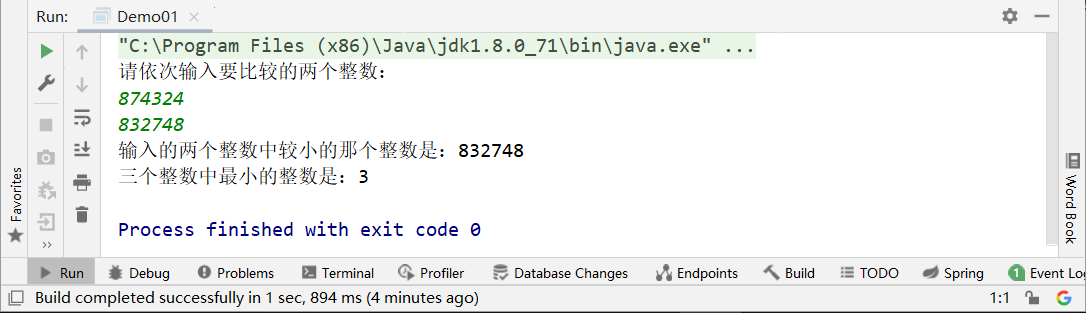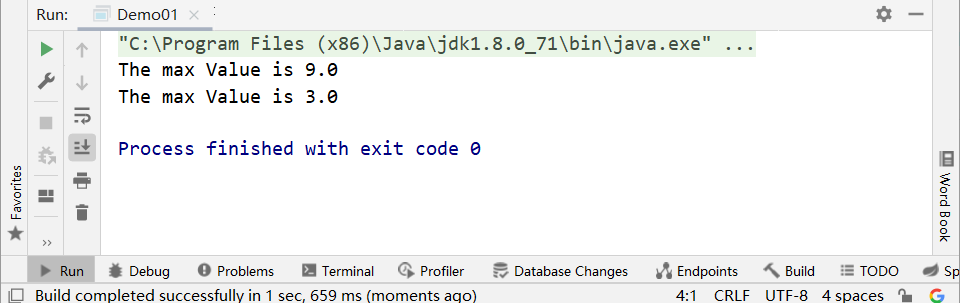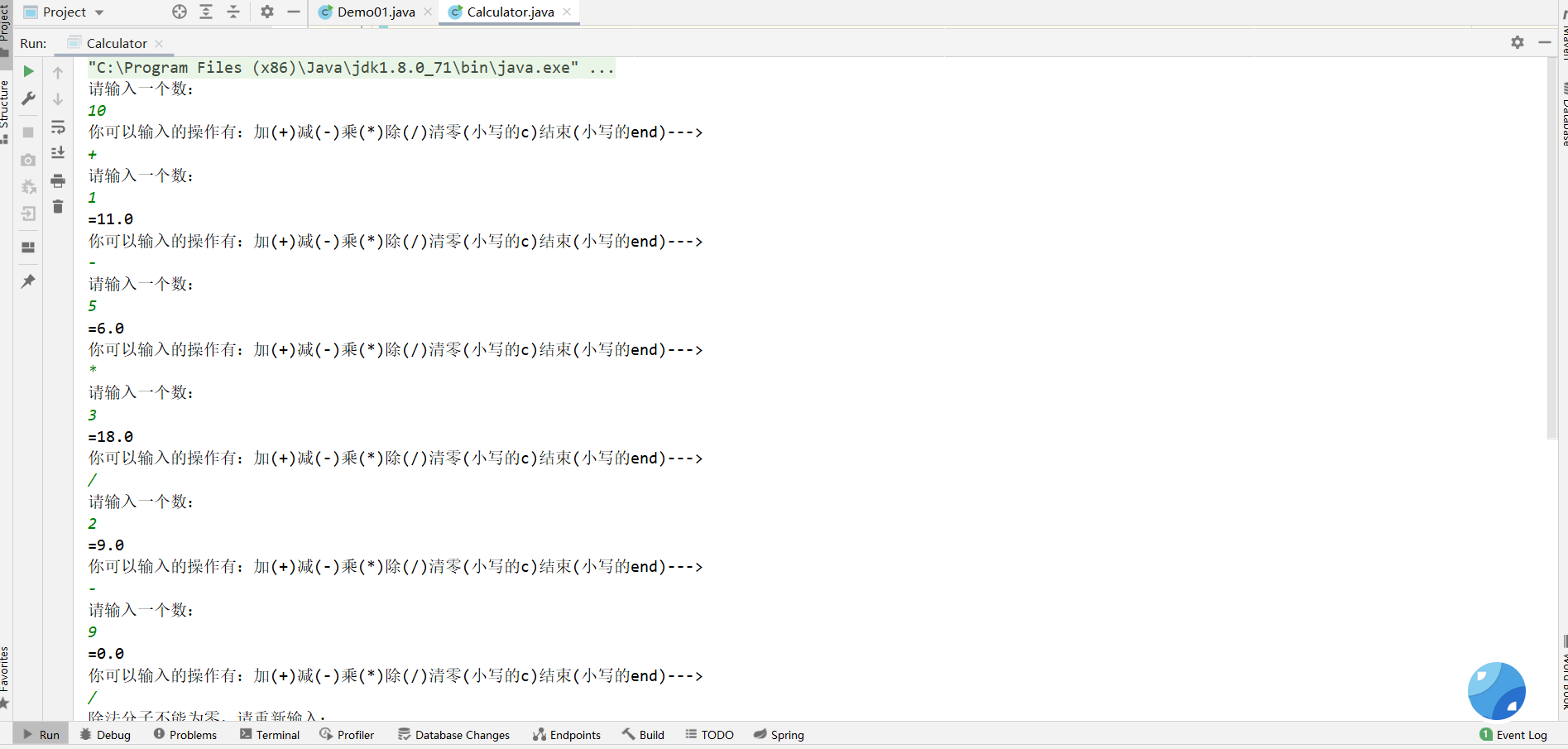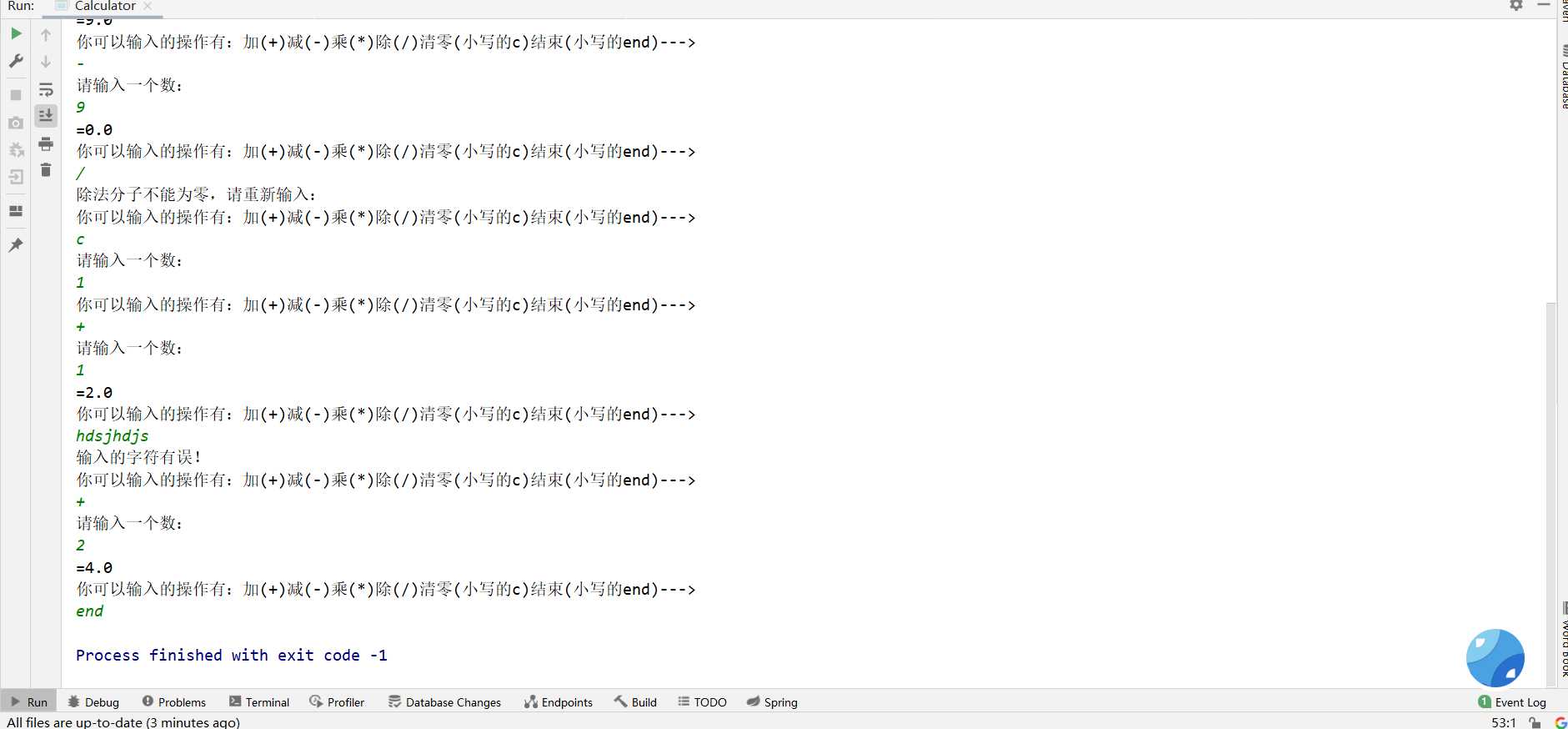# Java基础回顾_Java方法详解

deanyuancn

0收藏

Java基础回顾_Java方法详解

• Java方法是解决一类问题的步骤的有序组合
• 方法包含于类或对象中
• 方法在程序中被创建，在其它地方被引用

1. 方法命名遵循 小驼峰原则
2. main方法尽量保持简洁、干净
3. 方法其实就是C语言里的函数，方法遵循原子性，即一个方法只完成一件事
4. 有些方法没有return返回值，于是方法体就不需要写返回值类型，这个时候就要用 void 替代 返回值类型
5. 有参数的方法一定要有return返回值，没有参数的方法即void方法就不需要return返回值
6. 参数类型这里得注意：参数类型分为形式参数 和 实参（或称为变量）。

​ 形式参数：形参就是创建方法的时候我个人定义的像 int a, int b 这样的，没有实际值，等着调用的时候再往里传具体值的参数，相当于占位符，先把位置占着，用的时候再给它其他的值；

​ 实参（或称为变量）：方法在被调用的时候直接给参数赋值，像add( 1, 2);这种里面的 1和2 就是实参。

``````​ 修饰符 + 返回值类型 + 自定义方法名 + （ 参数类型 + 参数名--->”参数可以定义一个或者多个“ ）{

//执行的代码语句，即方法体

​ return + 返回值；

}

​ public int add( int a, int b ){

​ return a+b;

}``````

1、有时候用return 0；去终止方法。
2、方法的带你用：用对象名去点方法名，例如：对象名.方法名（实参列表）

Java都是值传递！只有值传递！

``````public class Demo01 {
public static void main(String[] args) {
Scanner scanner = new Scanner(System.in);
System.out.println("请依次输入要比较的两个整数：");
int a = scanner.nextInt();
int b = scanner.nextInt();
int min = min(a, b);
System.out.println("输入的两个整数中较小的那个整数是："+min);
int min1 = min(6, 8, 3);
System.out.println("三个整数中最小的整数是："+min1);
}
public static int min(int a,int b){
int minNumber;
if (a<b){
minNumber = a;
}else {
minNumber = b;
}
return minNumber;
}
//重载的取最小值的方法，但是参数列表不一样，多了一个参数
public static int min(int a,int b,int c){
int minNumber;
if (a<b){
minNumber = a;
}else {
minNumber = b;
}
if(minNumber<c){
return minNumber;
}else {
minNumber=c;
}
return minNumber;
}
}``````1、方法名称必须一模一样
2、参数列表必须不同，即参数的个数不同、或参数的类型不同、或参数的排列顺序不同等
3、方法的返回值类型可以相同，也可以不相同，但是仅仅只有返回值类型不同也不行

``````public class Demo01 {
public static void main(String[] args) {
printMax(1,5,3,4,6,8,2,3,9,6,4);
printMax(new double[]{1,2,3});
}
public static void printMax(double... numbers){
if (numbers.length == 0){
System.out.println("No argument passed");
return;
}
double result = numbers;

//排序！
for (int i = 1; i < numbers.length; i++) {
if (numbers[i] > result){
result = numbers[i];
}
}
System.out.println("The max Value is " + result);
}
}``````1. 递归头： 就是有一串可以停止调用方法（函数）的 代码，让程序不会无限循环运行！
2. 递归体：
友情提示：能不用递归就不用递归，因为如果调用方法次数过多，会非常占用资源，影响性能！

``````import java.util.Scanner;

public class Calculator{
public static void main(String[] args) {
//创建对象用来调用对象里的方法
Calculator calculator = new Calculator();
//调用computer方法
calculator.computer();
}
//第一步：加减乘除四个方法（因为要循环计算，每次传入一个数字，所以参数列表应该只有一个参数）
//加法
System.out.println("请输入一个数：");
Scanner scanner3 = new Scanner(System.in);
double b = scanner3.nextDouble();
return a+b;
}
//减法
public static double subtract(double a){
System.out.println("请输入一个数：");
Scanner scanner3 = new Scanner(System.in);
double b = scanner3.nextDouble();
return a-b;
}
//乘法
public static double multiply(double a){
System.out.println("请输入一个数：");
Scanner scanner3 = new Scanner(System.in);
double b = scanner3.nextDouble();
return a*b;
}
//除法
public static double divide(double a){
System.out.println("请输入一个数：");
Scanner scanner3 = new Scanner(System.in);
double b = scanner3.nextDouble();
return a/b;
}

//第二步：完成循环输入数据的功能（while（true）+switch）,必须创建一个方法完成这一步
public void computer(){
System.out.println("请输入一个数：");
Scanner scanner1 = new Scanner(System.in);
double a = scanner1.nextDouble();

while(true){
Scanner scanner2 = new Scanner(System.in);
System.out.println("你可以输入的操作有：加(+)减(-)乘(*)除(/)清零(小写的c)结束(小写的end)--->");
String str = scanner2.nextLine();
switch (str){
case "+":
System.out.println("="+a);
break;
case "-":
a = subtract(a);
System.out.println("="+a);
break;
case "*":
a = multiply(a);
System.out.println("="+a);
break;
case "/":
if (a == 0){
System.out.println("除法分子不能为零，请重新输入：");
break;
}
a = divide(a);
System.out.println("="+a);
break;
case "c":
computer();
break;
case "end":
System.exit(-1);
break;
default:
System.out.println("输入的字符有误！");
}
}
}

}
````````````int[] nums;//建议选择这种声明数组变量的格式；
int nums2[];//不建议使用这种格式；这是c和c++里的格式，早些年为了程序员能快速掌握Java所以也有这种声明数组变量的格式``````

``````int[] nums;//建议选择这种声明数组变量的格式；
int nums2[];//不建议使用这种格式；
nums = new int;//这里可以存放10个int类型的元素

//创建数组，一步到位
double[] nums3 = new double;
System.out.println(nums3.length);//输出nums3的数组长度``````

For-Each 循环

``int[][] a = {{1,2},{3,4},{8,9}};//这是一个二维数组``

Arrays类

Arrays.toString(数组名称)；//可以打印出数组所有数组元素；
Arrays.sort(数组名称)；//可以对数组排序：升序（从小到大）
Arrays.fill(数组名称,想要填充的值)；//可以对数组进行填充：所有元素都会被想要填充的值给替换
Arrays.fill(数组名称,3,8,想要填充的值)；//只有数组下标为3到8的元素被替换

``````public class Demo01 {
public static void main(String[] args) {
int[] array2 ={1,4,8,3,5,9,2};
System.out.println(Arrays.toString(fun1(array2  )));
}
//编写一个给数组冒牌排序的方法，参数为数组
public static int[] fun1(int[] array1){
//声明一个用来给两个相邻元素交换位置的变量
int t = 0;

for (int i = 0; i < array1.length-1; i++) {
for (int j = 0; j < array1.length-1-i; j++) {
if (array1[j+1]<array1[j]){
t = array1[j];
array1[j] = array1[j+1];
array1[j+1] = t;
}
}
}
return array1;``````

``````public class Demo01 {
public static void main(String[] args) {
//二维数组
int[][] array = new int;
array = 1;
array = 2;

for (int[] ints : array) {
for (int anInt : ints) {
System.out.print(anInt + "\t");
}
System.out.print("\n");
}
//将上面的二维数组转换为稀疏数组保存起来
//获取有效值的个数
int count = 0;
for (int i = 0; i < array.length; i++) {
for (int j = 0; j < array.length; j++) {
if(array[i][j] != 0){
count ++;
}
}
}
System.out.println("该二维数组中有效值的个数为："+ count);

//稀疏数组
//第一步：创建一个二维数组，称之为稀疏数组
int[][] array1 = new int[count+1];//有效值+1就是稀疏数组的行数，列固定为3列
//第二步，给这个稀疏数组加入第一行应该展示的数据；
array1 = array.length;//原数组的行数
array1 = array.length;//原数组的列数
array1 = count;//原数组中有效值 的个数

//第三步：给稀疏数组赋值
int count1 = 0;
for (int i = 0; i < array.length; i++) {
for (int j = 0; j < array[i].length; j++) {
if(array[i][j] != 0){
count1++;
array1[count1] = i;
array1[count1] = j;
array1[count1] = array[i][j];

}
}
}
//第四步：打印输出数组
System.out.println("原数组array的稀疏数组array1如下所示：");
//遍历输出稀疏数组array1
for (int[] ints : array1) {
for (int anInt : ints) {
System.out.print(anInt + "\t");
}
System.out.print("\n");
}
//        for (int i = 0; i < array1.length; i++) {
//            System.out.println(array1[i]+"\t"+array1[i]+"\t"+array1[i]);
//        }

System.out.println("将稀疏数组还原成原数组:");
//将稀疏数组还原成原数组
//第一步：用稀疏数组中第一行的值去创建一个二维数组，用来显示原数组
int array2[][] = new int[array1][array1];//确定数组为几行几列
//确认数组的大小后，就要通过稀疏数组中描述的原数组的有效值传入array2中
for (int i = 1; i < array1.length; i++) {
array2[array1[i]][array1[i]] = array1[i];
}
//第二步：打印array2数组
for (int[] ints : array2) {
for (int anInt : ints) {
System.out.print(anInt+"\t");
}
System.out.print("\n");
}
}

}``````

``````public class Demo01 {
public static void main(String[] args) {
//二维数组
int[][] array = new int;
array = 1;
array = 2;
System.out.println("数组array的稀疏数组为：" );
sparseArray(array);

int[][] array1 = new int;
array1 = 11;array1 = 11;array1 = 2;
array1 = 1;array1 = 2;array1 = 1;
array1 = 2;array1 = 3;array1 = 2;

System.out.println("稀疏数组array1的原数组为：" );
sparseArrayToArray(array1);

}

/**
* 数组 转为 稀疏数组
* @param array
*/
public static void sparseArray(int[][] array){
//将上面的二维数组转换为稀疏数组保存起来
//获取有效值的个数
int count = 0;
for (int i = 0; i < array.length; i++) {
for (int j = 0; j < array.length; j++) {
if(array[i][j] != 0){
count ++;
}
}
}
//稀疏数组
//第一步：创建一个二维数组，称之为稀疏数组
int[][] array1 = new int[count+1];//有效值+1就是稀疏数组的行数，列固定为3列
//第二步，给这个稀疏数组加入第一行应该展示的数据；
array1 = array.length;//原数组的行数
array1 = array.length;//原数组的列数
array1 = count;//原数组中有效值 的个数

//第三步：给稀疏数组赋值
int count1 = 0;
for (int i = 0; i < array.length; i++) {
for (int j = 0; j < array[i].length; j++) {
if(array[i][j] != 0){
count1++;
array1[count1] = i;
array1[count1] = j;
array1[count1] = array[i][j];

}
}
}
//第四步：打印输出数组

//遍历输出稀疏数组array1
for (int[] ints : array1) {
for (int anInt : ints) {
System.out.print(anInt + "\t");
}
System.out.print("\n");
}
}

/**
* 稀疏数组 转为 数组
* @param sparseArray
*/
public static void sparseArrayToArray(int[][] sparseArray){

//将稀疏数组还原成原数组
//第一步：用稀疏数组中第一行的值去创建一个二维数组，用来显示原数组
int array2[][] = new int[sparseArray][sparseArray];//确定数组为几行几列
//确认数组的大小后，就要通过稀疏数组中描述的原数组的有效值传入array2中
for (int i = 1; i < sparseArray.length; i++) {
array2[sparseArray[i]][sparseArray[i]] = sparseArray[i];
}
//第二步：打印array2数组
for (int[] ints : array2) {
for (int anInt : ints) {
System.out.print(anInt+"\t");
}
System.out.print("\n");
}
}

}
``````

1社区精华内容